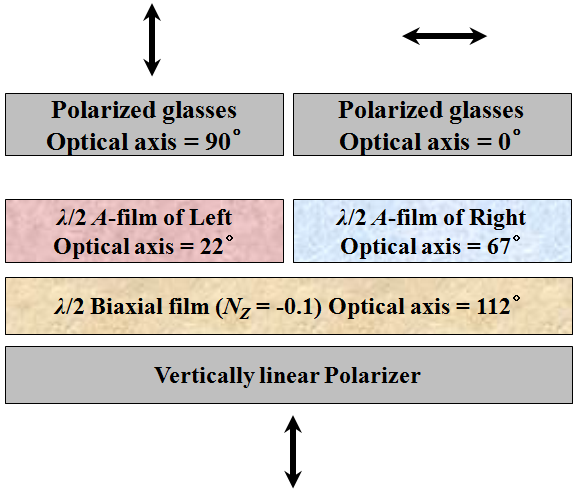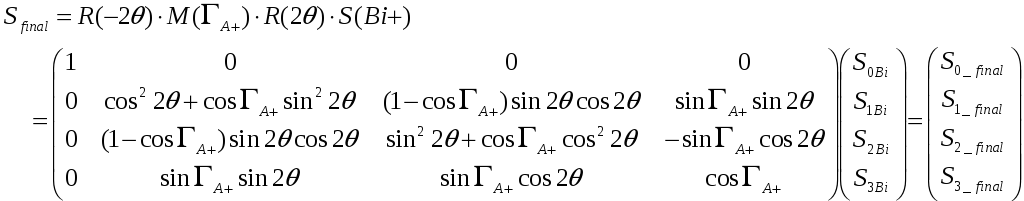# Optimal configuration for 3-d lc cell with half-wave retardation using Film Patterned Retarder technology

 Figure. 1. Conventional FPR system with the polarized glasses: (a) an optical structure and (b) the calculated light leakage for the right image in viewing angles. In order to completely separate the left and the right images in the normal and the oblique directions, we propose the optimal configuration for the FPR using wideband and wide-view half-wave retarder as shown in Fig. 2. The wide-band property of a proposed configuration can be achieved by satisfying wideband half-wave condition /2_1st layer film = /2_2nd layer film  45° and wide viewing angle property can be achieved by applying a half-wave biaxial film instead of a conventional half-wave A-film. In case of a biaxial film, it can produce a excellent optical property in the oblique direction because it has the parameter NZ [= (nx - nz) / (nx - ny)], which can control the deviation angle  by changing the NZ factor .Figure. 2. The proposed optimal configuration for a FPR system with the polarized glasses. We can calculate the changed polarization states after the incident light passes through each optical film in the normal and the oblique direction based on the Mueller matrix and the Stokes vector [14, 15]. The four Stokes parameters can usually be written as S = (S0, S1, S2, S3)T, and the Mueller matrix of a rotated retarder can be described as follows:where Sfinal represents the Stokes vector of the output light, S(Bi+) is the Stokes vector of the incident light after passing through the half-wave biaxial film, A+ is a retardation of the patterned positive A-film, R(2θ) and R(–2θ) are the rotating matrix and reverse-rotating matrix to the principal axis, respectively, and M(A+) depicts the Muller matrix for rotated polarizing components with phase retardation A+. In general, the polarization states of the light in front of the FPR system must obtain horizontally and vertically linear polarization, which is equal to Sfinal (1, 1, 0, 0) at the Stokes vector, in order to perform the excellent 3D image quality in a proposed FPR system. Thus, we use the parameter square method to find the optimized value of the film parameter that not only satisfy the wideband half-wave relationship and but also produce completely linear polarization states of the left and the right images at horizontal and vertical directions simultaneously. Figure 3 shows a two-dimensional parameter space map for the optimization of the FPR 3D structure. We set the optimization range with the S1 value between 0.994 and 1. From optimization of the half-wave biaxial film, the optical axis of 112° and the NZ factor of -0.1 can be obtained in horizontal and vertical direction.Share with your friends: